Learning focus

In this lesson, you will learn how to add two three-digit numbers together.

This lesson includes:

• one quiz
• one learning summary
• one video
• two practise activities

Quiz

Check your knowledge of adding two 3-digit numbers together in this quiz. Get your pen and paper handy and try using the column method.

Learn

First, let’s find out a bit about the method for adding numbers together using number columns. Watch this short animation featuring a hive of helpful bees from KS2 Maths.

Checklist

There are a few things you should always do when adding large numbers together:

• When writing down sums, separate the numbers into ones, tens and hundreds. List the numbers in a column and always start adding with the ones first.
• Estimate first and check afterwards - it's a good idea to estimate a rough answer first and then check your actual answer.
• Order doesn't matter - remember that 345 + 129 is the same as 129 + 345.
• Key words - look out for these words in problems: total, sum, altogether, more. They all indicate an addition calculation.

Numbers can be added together using the column method which involves writing one number underneath the other.

Example 1

What is 343 + 315?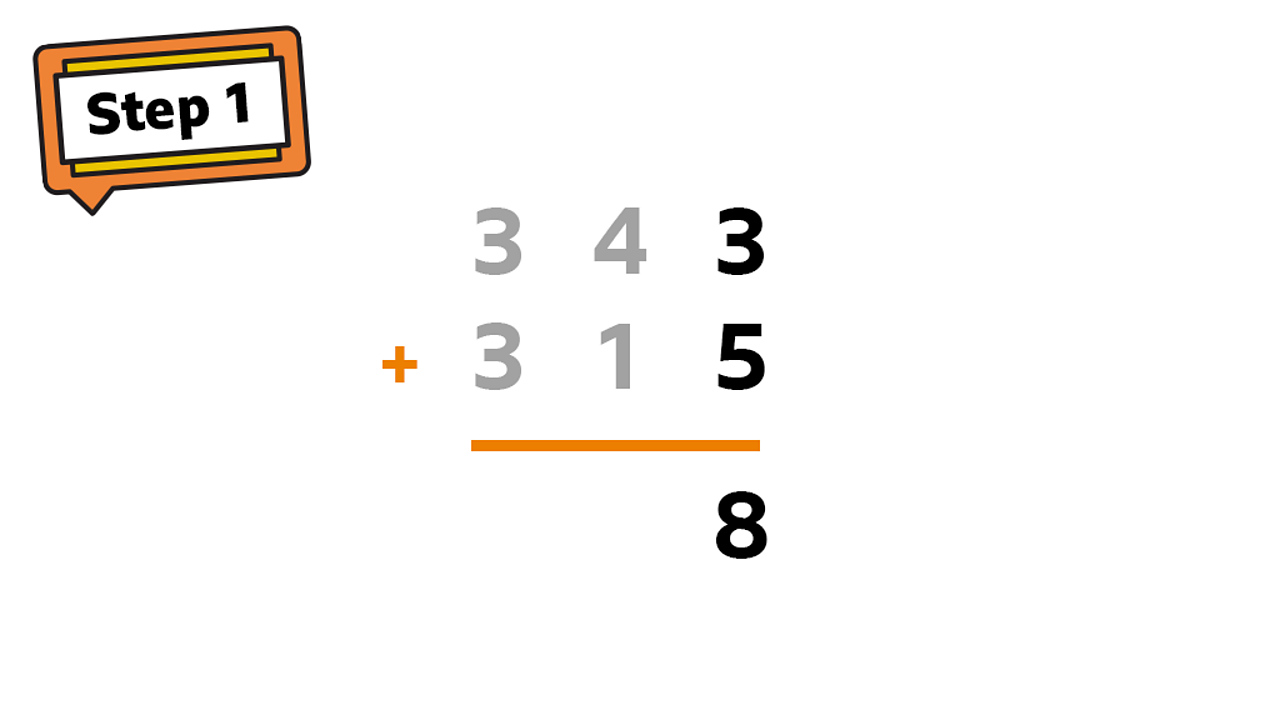Start from the ones column: 3 + 5 = 8.1 of 4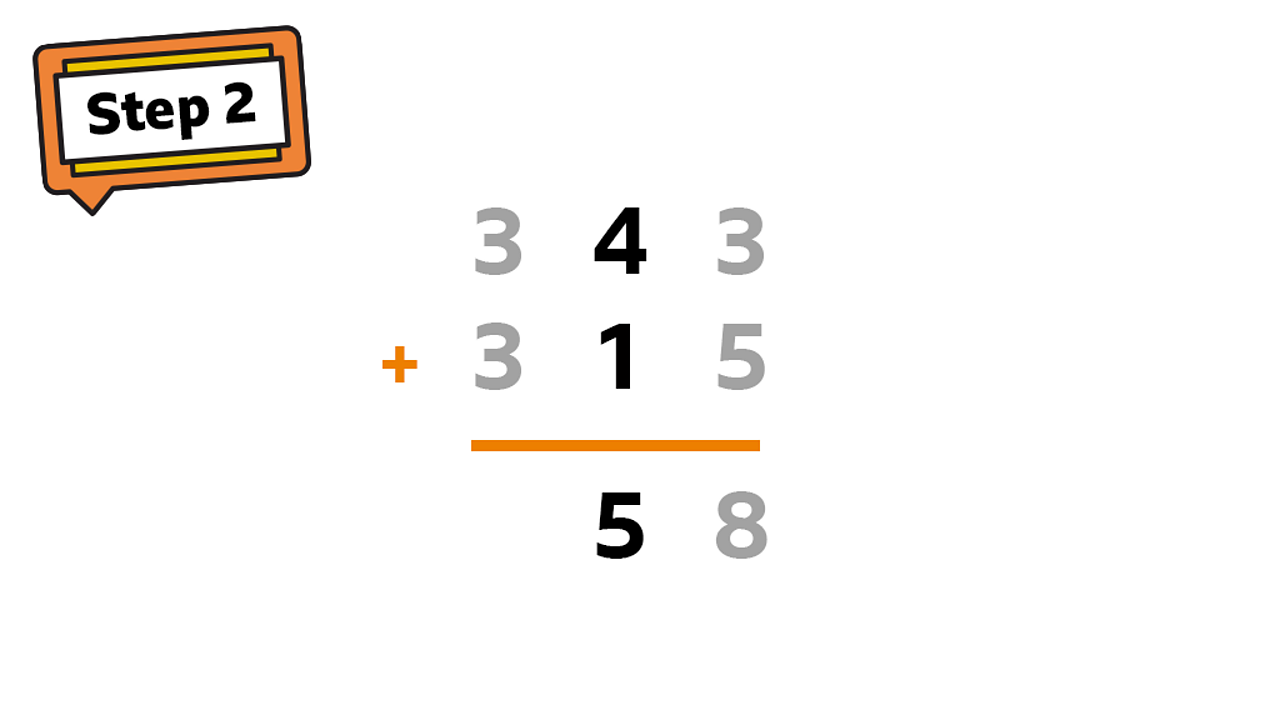In the tens column: 40 + 10 = 50.2 of 4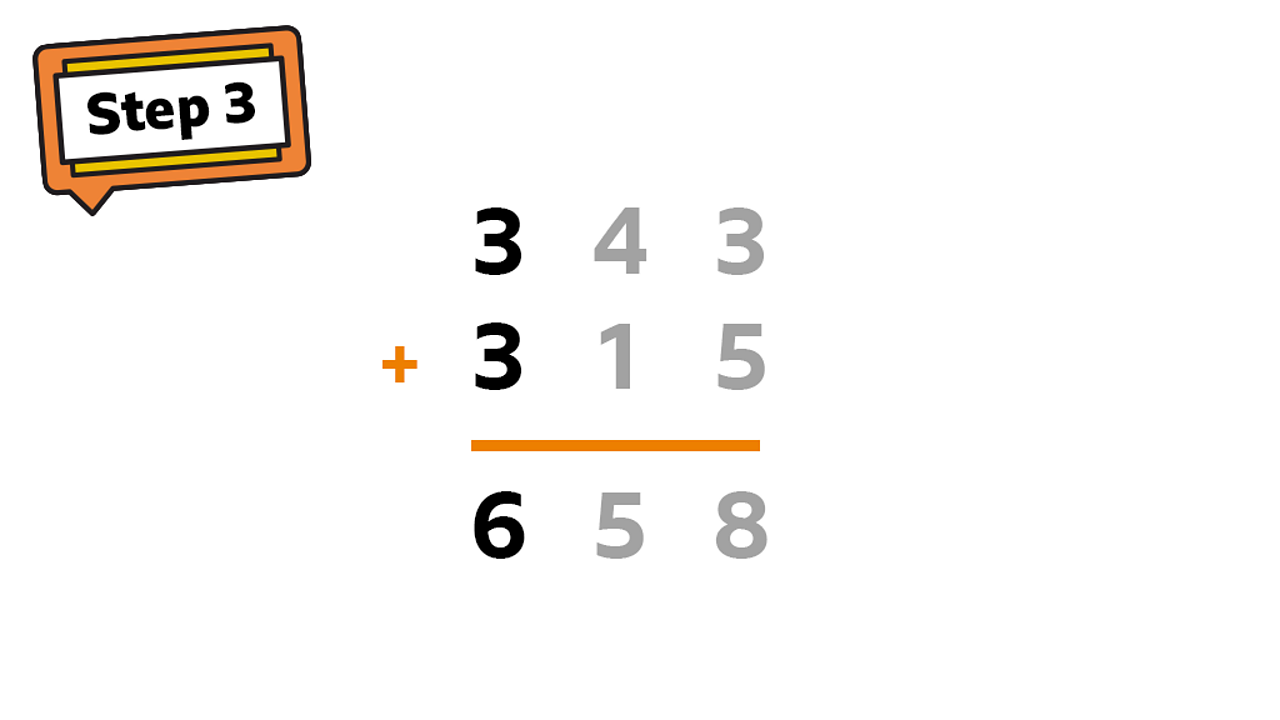In the hundreds column: 300 + 300 = 600.3 of 4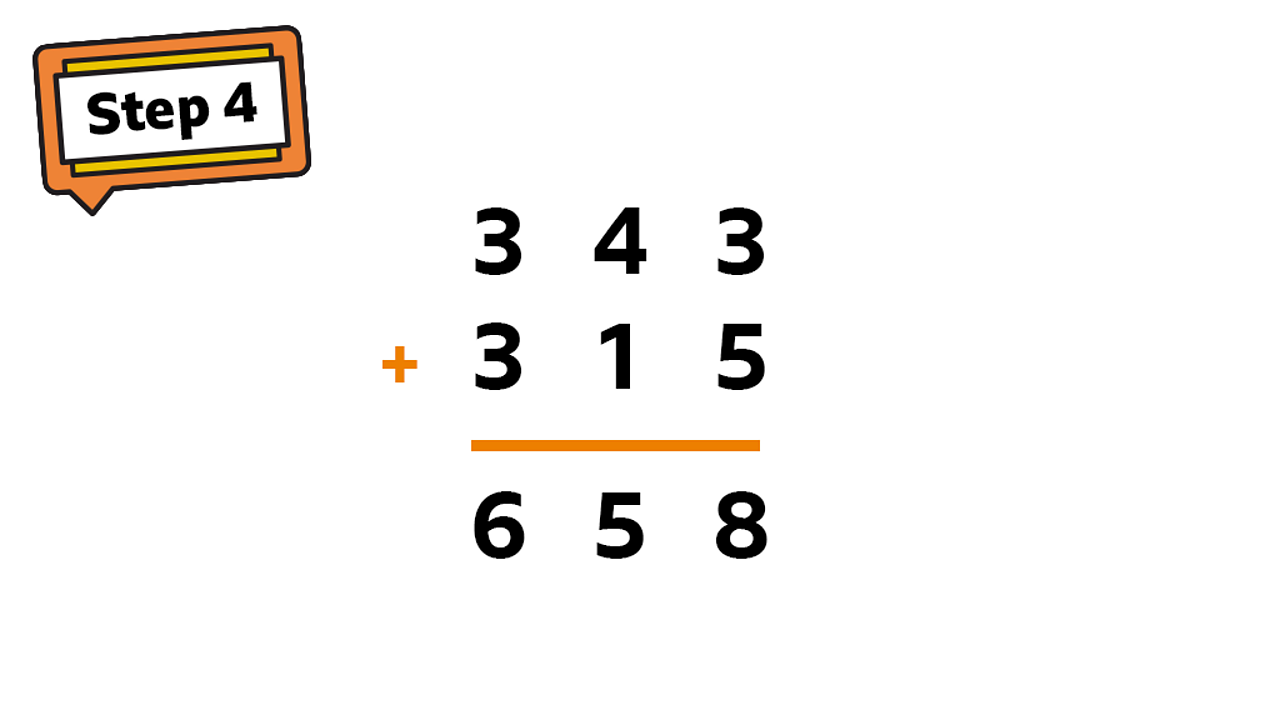The finished sum. 343 + 315 = 658.4 of 4

Example 2

What is 343 + 329?

If the two numbers in a place value column add up to a number greater than 9, you must carry a digit.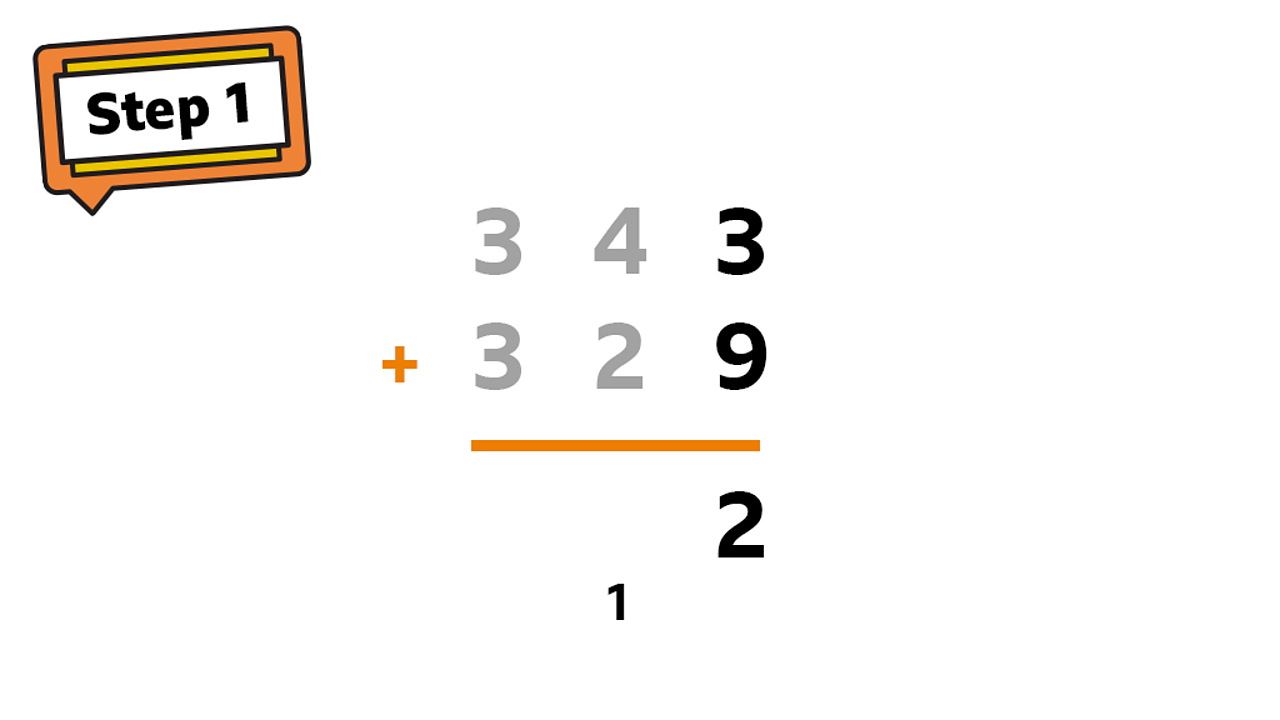Start from the ones column: 3 + 9 = 12. Write a 2 in the ones column and carry 10 to the tens column by writing a 1 below.1 of 4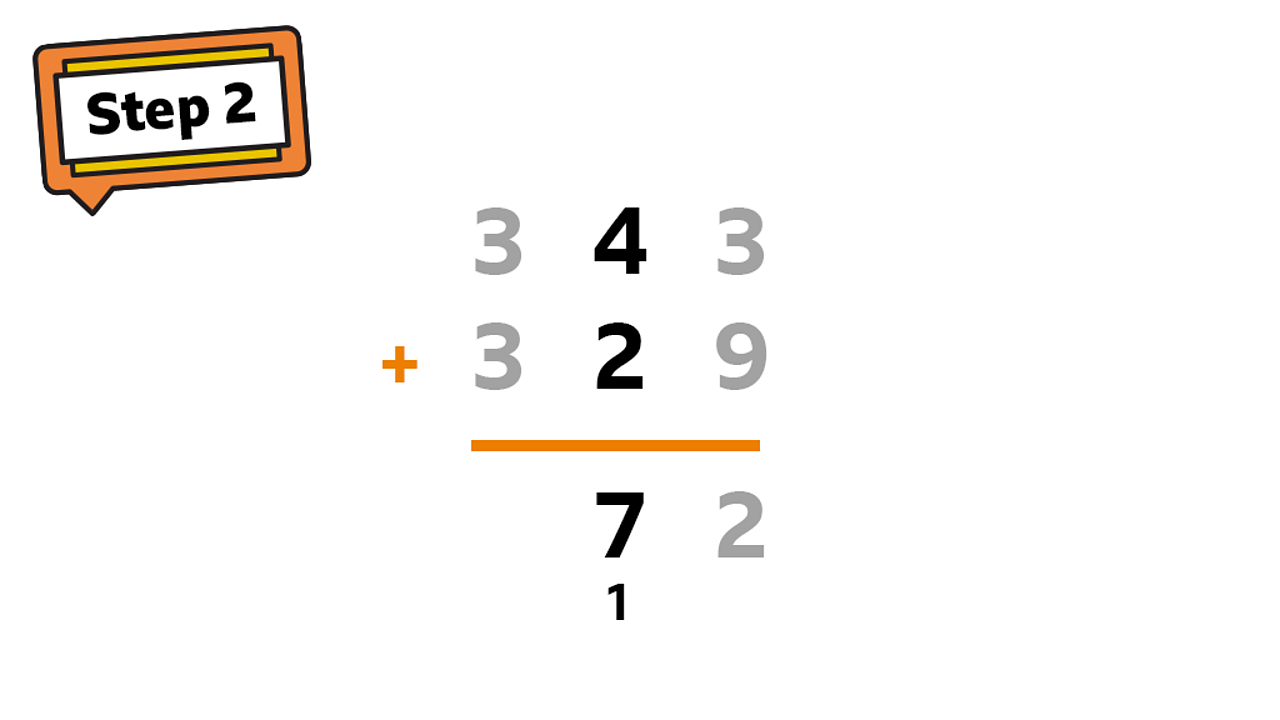In the tens column: 40 + 20 + 10 = 70. Remember to add that extra ten you carried over.2 of 4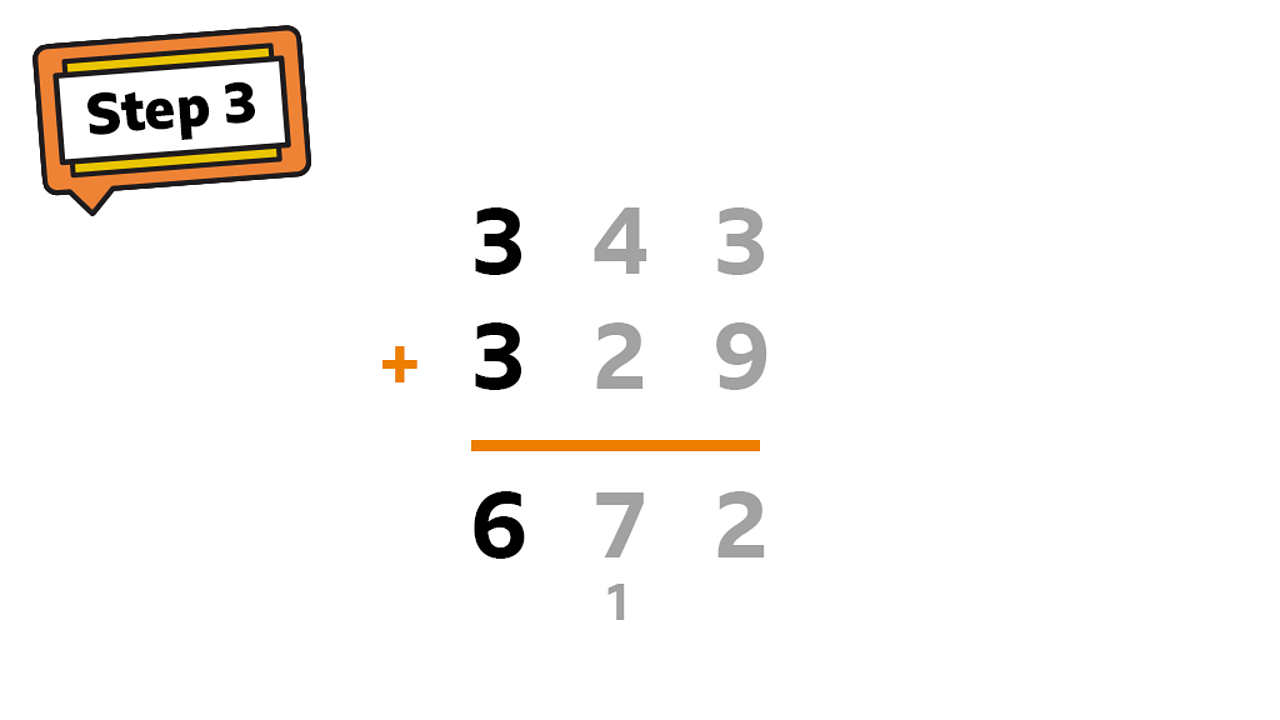In the hundreds column: 300 + 300 = 600.3 of 4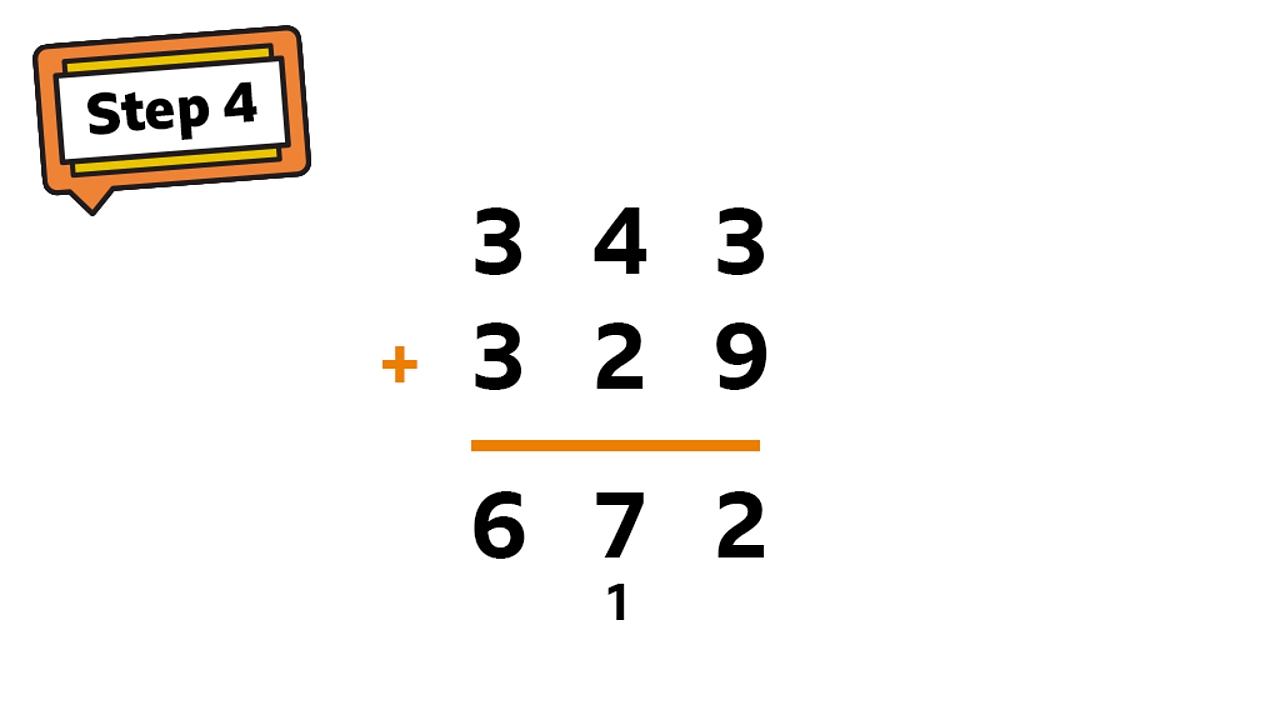The finished sum. 343 + 329 = 672.4 of 4

Example 3

One apple tree has 178 apples. Another has 253 apples. How many apples are there altogether?

You can use the column method to solve this problem.

Practise

Activity 1

Hive maths

Click on the image below to see if you can add the numbers correctly.

Activity 2

On a piece of paper, and using the column method, try to work out the following:

1. 258 + 351

2. 541 + 639

3. 745 + 362